# BITSAT Mathematics Trigonometric Functions MCQs

BITSAT Mathematics Trigonometric Functions MCQs with answers available in Pdf for free download. The MCQ Questions for BITSAT Mathematics with answers have been prepared as per the latest BITSAT Mathematics syllabus, books and examination pattern. Multiple Choice Questions form important part of competitive exams and BITSAT exam and if practiced properly can help you to get higher rank. Refer to more topic wise BITSAT Mathematics Questions and also download more latest study material for all subjects and do free BITSAT Mathematics Mock Test

## MCQ for BITSAT Mathematics Trigonometric Functions

BITSAT Mathematics students should refer to the following multiple-choice questions with answers for Trigonometric Functions in BITSAT. These MCQ questions with answers for BITSAT Mathematics will come in exams and help you to score good marks

### Trigonometric Functions MCQ Questions with Answers

• a) 4
• b) 5
• c) 6
• d) 8

#### Question: If A and B are positive acute angles satisfying, Then the value of A + 2B is equal to :

• a)• b)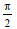• c)• d)• a) 0
• b) 1
• c) 2
• d) 3

#### Question: If tan (cot x) = cot (tan x), then sin 2x is equal to :

• a)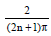• b)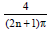• c)• d)#### Question: The general solution of the equation sin 2x + 2sin x +2 cos x+ 1 = 0 is

• a)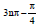• b)• c)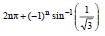• d)• a) 1 + n2
• b) 1 – n2
• c) n2
• d) – n2

#### Question: The period of tan 3θ is

• a) π
• b) 3π/4
• c) π/2
• d) None of these

• a) 0
• b) 1
• c) 1/6
• d) 6

#### Question: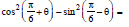• a)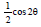• b) 0
• c)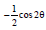• d)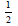#### Question: The solution of (2 cos x – 1) (3 + 2 cos x) = 0 in the interval• a)• b)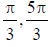• c)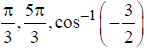• d) None of these#### Question: If x sin3 θ + ycos3 θ = sin θcos θ and x sinθ = ycos θ , then x2 + y2 =

• a) 1
• b) 2
• c) 0
• d) None of these

#### Question: If cos 7θ=cosq - sin 4θ, then the general value of θ is

• a)• b)• c)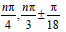• d)• a) 3/8
• b) 5/8
• c) 3/4
• d) 5/4

#### Question: If, tanand tan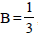, then find the value of A + B

• a) π
• b)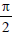• c)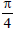• d)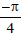#### Question: If sin θ=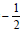and tan θ = 1/ √3 then θ =

• a) 2nπ + π/6
• b) 2nπ +11π/6
• c) 2nπ +7π/6
• d) 2nπ + π/4

#### Question: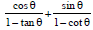is equal to

• a) sinθ – cos θ
• b) sinθ + cosθ
• c) tanθ + cotθ
• d) tanθ – cot θ

#### Question: If 12cot2 θ - 31cosecθ + 32 = 0, then the value of sin θ is

• a)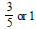• b)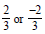• c)• d)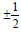• a) √3 / 2
• b) √3 / 4
• c) √3
• d) 1

#### Question: If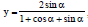then value ofis

• a)• b) y
• c) 2y
• d)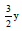#### Question: Period of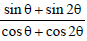is

• a) 2π
• b) π
• c)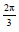• d)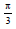#### Question: The general solution of 8tan2+ sec x is

• a)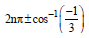• b)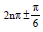• c)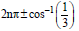• d) None of these• a) √3
• b) √2
• c) 1
• d) 2

#### Question: The value of tan A + tan(60° + A) - tan(60° - A) is

• a) tan 3A
• b) 2 tan 3A
• c) 3 tan 3A
• d) None of these

#### Question: If tan θ =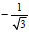, then the general solution of the equation

• a)• b)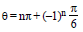• c)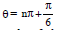• d)• a) 1
• b) 2
• c) 3
• d) 4

#### Question: If A = cos2 θ+sin4 θ, then for all real values of θ

• a)• b)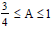• c)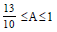• d)#### Question: If sin θ+ cosecθ = 2, then sin2 θ + cosec 2θ is equal to

• a) 1
• b) 4
• c) 2
• d) None of these

#### Question: If cot x – cos x cot x = 1 – cos x then general solution of this equation is

• a)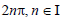• b)• c)• d)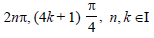#### Question: If sec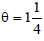then• a)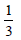• b)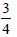• c)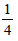• d)#### Question: If 2θ cosθ = xsin θ and 2xsecθ- y cosec θ = 3, then x2 + 4y2 =

• a) 4
• b) -4
• c)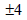• d) None of these